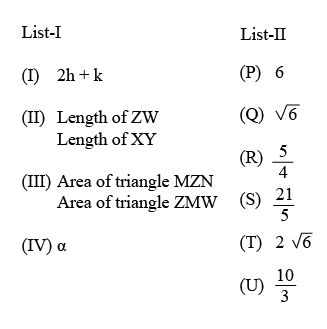### JEE (Advanced) 2019 Paper-2 Question 54

Instructions

Answer by appropriately matching the lists based on the information given in the paragraph.
Let the circles $$C_1 : x^2 + y^2 = 9$$ and $$C_2 : (x - 3)^2 + (y - 4)^2 = 16,$$ intersect at the points X and Y. Suppose that another circle $$C_3 : (x - h)^2 + (y - k)^2 = r^2$$ satisfies the following conditions:
(i) centre of $$C_3$$ is collinear with the centres of $$C_1$$ and $$C_2$$,
(ii) $$C_1$$ and $$C_2$$ both lie inside $$C_3$$ and
(iii) $$C_3$$ touches $$C_1$$ at M and $$C_2$$ at N.
Let the line through X and Y intersect $$C_3$$ at Z and W, and let a common tangent of $$C_1$$ and $$C_3$$ be a tangent to the parabola $$x^2 = 8 \alpha y.$$
There are some expressions given in the List-I whose values are given in List-II below:Question 54